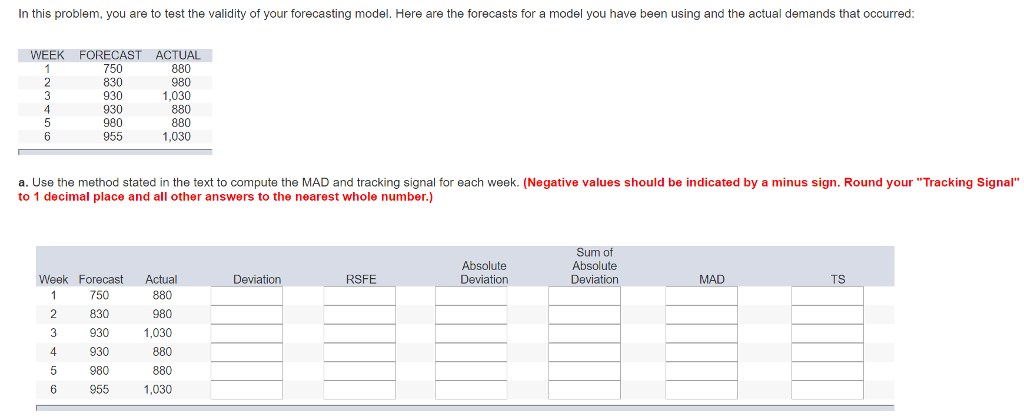# Question & Answer: In this problem, you are to test the validity of your forecasting model. Here are the forecasts for…..In this problem, you are to test the validity of your forecasting model. Here are the forecasts for a model you have been using and the actual demands that occurred: a. Use the method stated in the text to compute the MAD and tracking signal for each week. (Negative values should be indicated by a minus sign. Round your “Tracking Signal” to 1 decimal place and all other answers to the nearest whole number.)